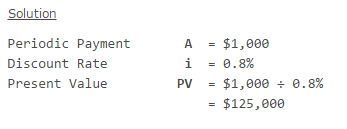Accounting

# Present Value of Perpetuity Mathematical Explanation

Perpetuity is an infinite series of periodic payments of equal face value. In other words, perpetuity is a situation where a constant payment is to be made periodically for an infinite amount of time. It as an annuity having no end and that is why the perpetuity is sometimes called as perpetual annuity.

Although the total face value of perpetuity is infinite and undeterminable, its present value is not. According to the time value of money principle, the present value of perpetuity is the sum of the discounted value of each periodic payment of the perpetuity. Present value of perpetuity is finite because the discounted value of far future payments of the perpetuity reduces considerably and reaches close to zero.

Formula

The following formula is used to calculate the present value of perpetuity:

Present Value (PV) of Perpetuity: A/r

Where,
A is the fixed periodic payment; and
r is the interest rate or discount rate per compounding period.

Example:

Calculate the present value on Jan 1, 20X0 of a perpetuity paying \$1,000 at the end of each month starting from January 20X0. The monthly discount rate is 0.8%.